×#### Thank you for registering.

One of our academic counsellors will contact you within 1 working day.

Click to Chat

1800-1023-196

+91-120-4616500

CART 0

• 0

MY CART (5)

Use Coupon: CART20 and get 20% off on all online Study Material

ITEM
DETAILS
MRP
DISCOUNT
FINAL PRICE
Total Price: Rs.

There are no items in this cart.
Continue Shopping• Complete JEE Main/Advanced Course and Test Series
• OFFERED PRICE: Rs. 15,900
• View Details

```Chapter 1: Fractions Exercise – 2.2

Question: 1

Multiply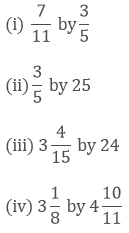Solution:

(i)  We have,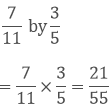(ii)  We have,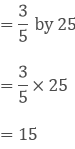(iii)  We have,(iv) We have,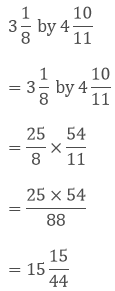Question: 2

Find the product: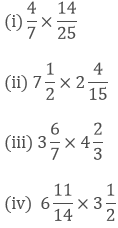Solution:

(i)  We have,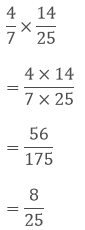(ii) We have,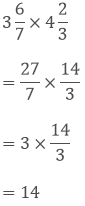(iv) We have,Question: 3

Simplify: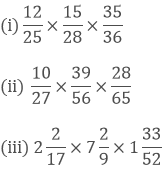Solution:

(i)  We have,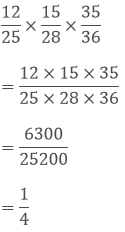(ii) We have,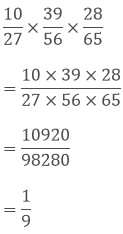(iii) We have,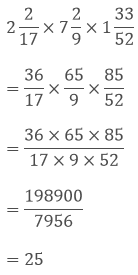Question: 4

Find: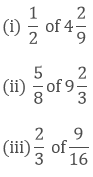Solution:

(i) We have,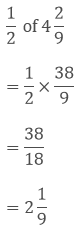(ii) We have,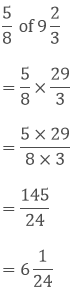(iii) We have,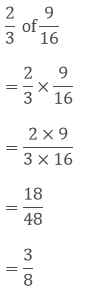Question: 5

Which is greater?Solution:While comparing two fractions, when the numerators of both the fractions are same, then the denominator having higher value shows the fraction has lower value.

So, 6/14 is greater.

Therefore, 1/2 of 6/7 is greater.

Question: 6

(i)  7/11 of 330

(ii) 5/9 of 108 meters

(iii) 3/7 of 42 litres

(iv) 1/12 of an hour

(v) 5/6 of an year

(vi) 3/20 of a Kg

(vii) 7/20 of a litres

(viii) 5/6 of a day

(ix)  2/7 of a week

Solution:

(i)  We have,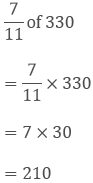(ii)  We have,

5/9 of 108 meters

= 5/9 × 108 meters

= 5 × 12 meters

60 meters

(iii) We have,

3/7 of 42 litres

= 3/7× 42 litres

= 3 × 6 litres

=18 litres

(iv) We have,

1/12 of an hour

An hour = 60 minutes

Therefore, 1/12 × 60 minutes

= 5 minutes

(v) We have,

5/6 of an year

I Year = 12 months

Therefore,

5/6 × 12 months

= 5 × 2 months

= 10 months

(vi) We have,

3/20 of a kg

1 Kg = 1000 gms

Therefore,

3/20 × 1000 gms

= 3 × 50 gms

= 150 gms

(vii) We have,

7/20 of a litre

1 litre = 1000 ml

Therefore,

7/20 × 1000 ml

= 7 × 50 ml

= 350 ml

(viii) We have,

5/6 of a day

I day = 24 hours

Therefore,

5/6 × 24 hours

= 5 × 4 hours

= 20 hours

(ix) We have,

2/7 of a week

I week = 7 days

Therefore,

2/7 × 7 days = 2 days

Question: 7

Shikha plans 5 saplings in a row in her garden. The distance between two adjacent saplings is 3/4 m. Find the distance between first and last sapling.

Solution:

There are 4 adjacent spacing for 5 saplings.

Given, the distance between two adjacent saplings is 3/4 m.

4 adjacent spacing for 5 saplings = 3/4 × 4 = 3 m

Therefore, the distance between first and last sapling is 3 m.

Question: 8

Ravish reads 1/3 part of a book in one hour. How much part of the book will be read in 2(1/5) hours?

Solution:

Let x be the full part of book.

Given, Ravish reads 1/3 part of a book in one hour

1 hour = (1/3) x

Part of the book will he read in 2(1/5) hours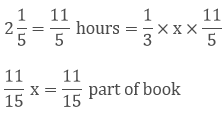Question: 9

Lipika reads a book for 1(3/4) hours every day. She reads the entire book in 6 days. How many hours in all were required by her to read the book?

Solution:

Given,

Time taken by Lipika to read a book per day = 1(3/4) = 7/4 hours

Time taken by Lipika to read a book for 6 days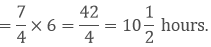Question: 10

Find the area of a rectangular park which is 41(2/3)m long and 18(3/5)m broad.

Solution: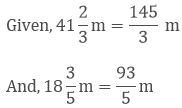Area of a rectangular park = (length x breadth)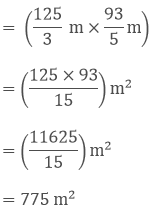Question: 11

If milk is available at Rs 17(3/4) per litre, find the cost of 7(2/5) litres of milk.

Solution:

Given,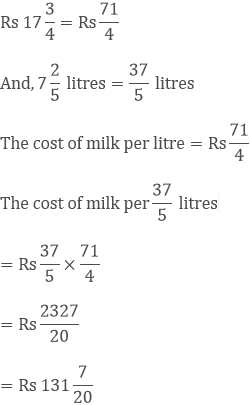Question: 12

Sharda can walk 8(1/3) km in one hour. How much distance will she cover in 2(2/5) hours.

Solution:

Given,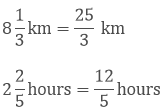Distance covered by Sharda in one hour = 25/3 km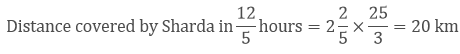Question: 12

A sugar bag contains 30 kg of sugar. After consuming 2/3 of it, how much sugar is left in the bag

Solution:

Given, A sugar bag contains 30 kg of sugar

After consuming 23 of it, the amount of sugar left in the bagQuestion: 14

Each side of a square is 6 (2/3)m long. Find its area.

Solution:

Given,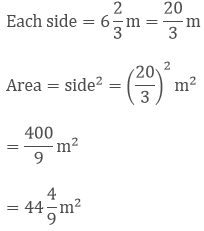Question: 15

There are 45 students in a class and 3/5 of them are boys. How many girls are. there in the class?

Solution:

Given,

There are 45 students in a class,

And 3/5 of them are boys.

Therefore, no of girls in the class = 45 –3/5 × 45

= 45 – 27

= 18
```### Course Features

• 728 Video Lectures
• Revision Notes
• Previous Year Papers
• Mind Map
• Study Planner
• NCERT Solutions
• Discussion Forum
• Test paper with Video Solution